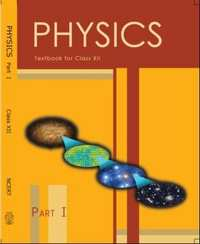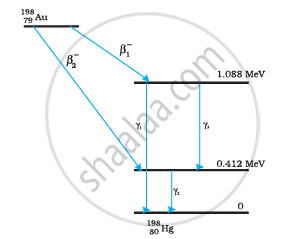Share

# NCERT solutions for Class 12 Physics Textbook chapter 13 - Nuclei [Latest edition]

Textbook page

#### Chapters## Chapter 13: Nuclei

#### NCERT solutions for Class 12 Physics Textbook Chapter 13 Nuclei [Pages 462 - 466]

Q 1.1 | Page 462

Two stable isotopes of lithium ""_3^6Li and ""_3^7Li have respective abundances of 7.5% and 92.5%. These isotopes have masses 6.01512 u and 7.01600 u, respectively. Find the atomic mass of lithium.

Q 1.2 | Page 462

Boron has two stable isotopes, ""_5^10B and Their respective masses are  10.01294 u and 11.00931 u, and the atomic mass of boron is 10.811 u. Find the abundances of  ""_5^10B  and ""_5^11B.

Q 2 | Page 462

The three stable isotopes of neon: ""_10^20Neand ""_10^22 Nehave respective abundances of 90.51%, 0.27% and 9.22%. The atomic masses of the three isotopes are 19.99 u, 20.99 u and 21.99 u, respectively. Obtain the average atomic mass of neon

Q 3 | Page 462

Obtain the binding energy (in MeV) of a nitrogen nucleus(""_7^14N), given m(""_7^14N)=14.00307 u

Q 4 | Page 462

Obtain the binding energy of the nuclei ""_56^26Fe and ""_83^209Biin units of MeV from the following data:

m(""_26^56Fe) = 55.934939 u m(""_83^209Bi)= 208.980388 u

Q 5 | Page 462

A given coin has a mass of 3.0 g. Calculate the nuclear energy that would be required to separate all the neutrons and protons from each other. For simplicity assume that the coin is entirely made of ""_29^63Cuatoms (of mass 62.92960 u).

Q 6.1 | Page 462

Write nuclear reaction equations for  α-decay of ""_88^226Ra

Q 6.2 | Page 462

Write nuclear reaction equations for α-decay of ""_94^242Pu

Q 6.3 | Page 462

Write nuclear reaction equations for β-decay of ""_15^32P

Q 6.4 | Page 462

Write nuclear reaction equations for β-decay of ""_83^210Bi

Q 6.5 | Page 462

Write nuclear reaction equations for β+-decay of ""_6^11C

Q 6.6 | Page 462

Write nuclear reaction equations for β+-decay of ""_43^97Tc

Q 6.7 | Page 462

Write nuclear reaction equations for Electron capture of ""_54^120Xe

Q 7 | Page 462

A radioactive isotope has a half-life of years. How long will it take the activity to reduce to a) 3.125%, b) 1% of its original value?

Q 8 | Page 462

The normal activity of living carbon-containing matter is found to be about 15 decays per minute for every gram of carbon. This activity arises from the small proportion of radioactive ""_6^14C present with the stable carbon isotope ""_6^12C . When the organism is dead, its interaction with the atmosphere (which maintains the above equilibrium activity) ceases and its activity begins to drop. From the known half-life (5730 years) of ""_6^14C and the measured activity, the age of the specimen can be approximately estimated. This is the principle of ""_6^14C" dating used in archaeology. Suppose a specimen from Mohenjodaro gives an activity of 9 decays per minute per gram of carbon. Estimate the approximate age of the Indus-Valley civilisation.

Q 9 | Page 463

Obtain the amount of ""_27^60Co necessary to provide a radioactive source of 8.0 mCi strength. The half-life of ""_27^60Co is 5.3 years

Q 10 | Page 463

The half-life of ""_38^90 Sr is 28 years. What is the disintegration rate of 15 mg of this isotope?

Q 11 | Page 463

Obtain approximately the ratio of the nuclear radii of the gold isotope ""_97^197 Au and the silver isotope ""_47^197Ag.

Q 12 | Page 463

Find the Q-value and the kinetic energy of the emitted α-particle in the α-decay of

a) ""_88^226 Ra and  b) ""_86^220Rn

Given m(""_88^226Ra) = 226.02540 u, m(""_86^222 Rn) = 222.01750 u,

m(""_86^220 Rn)= 220.01137 u, m(""_84^216 Po)= 216.00189 u.

Q 13 | Page 463

The radionuclide 11C decays according to ""_6^11C ->  _5^11B + e^+ + v:"   T_(1/2) = 20.3 min

The maximum energy of the emitted positron is 0.960 MeV.

Given the mass values: m(""_6^11C) = 11.011434 u and m(""_6^11B) = 11.009305 u

calculate and compare it with the maximum energy of the positron emitted

Q 14 | Page 463

The nucleus ""_10^23Ne decays by beta^(-)emission. Write down the β decay equation and determine the maximum kinetic energy of the electrons emitted. Given that:

m(""_10^23 Ne) = 22.994466 u

m(""_11^23 Na) = 22.989770 u.

Q 15.1 | Page 463

The value of a nuclear reaction → is defined by

= [ mAmb− mC− md]cwhere the masses refer to the respective nuclei. Determine from the given data the Q-value of the following reactions and state whether the reactions are exothermic or endothermic.

""_1^1H + _1^3H -> _1^2H + _1^2H

Atomic masses are given to be

m(""_1^2H) = 2.014102 u

m(""_1^3H) = 3.016049 u

m(""_6^12C) = 12.000000 u

m(""_10^20Ne) = 19.992439 u

Q 15.2 | Page 463

The Q value of a nuclear reaction A + b → C + d is defined by

Q = [ mA+ mb− mC− md]c2 where the masses refer to the respective nuclei. Determine from the given data the Q-value of the following reactions and state whether the reactions are exothermic or endothermic.

""_6^12C + _6^12C -> _10^20Ne + _2^4He

Atomic masses are given to be

m(""_1^2H) = 2.014102 u

m(""_1^3H) = 3.016049 u

m(""_6^12C) = 12.000000 u

m(""_10^20Ne) = 19.992439 u

Q 16 | Page 463

Suppose, we think of fission of a ""_26^56Fe nucleus into two equal fragments ""_13^28Al. Is the fission energetically possible? Argue by working out of the process. Given  m(""_26^56 Fe) = 55.93494 u  and m(""_13^28 Al) = 27.98191 u.

Q 17 | Page 463

The fission properties of ""_94^239Pu are very similar to those of ""_92^235 U.

The average energy released per fission is 180 MeV. How much energy, in MeV, is released if all the atoms in 1 kg of pure ""_94^239 Pu undergo fission?

Q 18 | Page 464

A 1000 MW fission reactor consumes half of its fuel in 5.00 y. How much ""_92^235U did it contain initially? Assume that the reactor operates 80% of the time, that all the energy generated arises from the fission of ""92^235U and that this nuclide is consumed only by the fission process.

Q 19 | Page 464

How long can an electric lamp of 100W be kept glowing by fusion of 2.0 kg of deuterium? Take the fusion reaction as

""_1^2H + _1^2H -> _1^3He + n +  3.27 MeV

Q 20 | Page 464

Calculate the height of the potential barrier for a head on collision of two deuterons.

(Hint: The height of the potential barrier is given by the Coulomb repulsion between the two deuterons when they just touch each other. Assume that they can be taken as hard spheres of radius 2.0 fm.)

Q 21 | Page 464

From the relation R0A1/3, where R0 is a constant and is the mass number of a nucleus, show that the nuclear matter density is nearly constant (i.e. independent of A).

Q 22 | Page 464

For the beta^+ (positron) emission from a nucleus, there is another competing process known as electron capture (electron from an inner orbit, say, the K−shell, is captured by the nucleus and a neutrino is emitted).

e^+ + ""_Z^A X -> _(Z-1)^A Y + v

Show that if beta^+ emission is energetically allowed, electron capture is necessarily allowed but not vice−versa.

Q 23 | Page 464

In a periodic table the average atomic mass of magnesium is given as 24.312 u. The average value is based on their relative natural abundance on earth. The three isotopes and their masses are ""_12^24Mg (23.98504u),  ""_12^25 Mg (24.98584u) and ""_12^26Mg (25.98259u). The natural abundance of ""_12^24 Mg is 78.99% by mass. Calculate the abundances of other two isotopes.

Q 24 | Page 464

The neutron separation energy is defined as the energy required to remove a neutron from the nucleus. Obtain the neutron separation energies of the nuclei ""_20^41Ca and ""_13^27 Al from the following data:

m(""_20^40Ca) = 39.962591 u

m(""_20^41Ca) = 40.962278 u

m(""_13^26Al) = 25.986895  u

m(""_13^27Al) = 26.981541 u



Q 25 | Page 464

A source contains two phosphorous radio nuclides ""_15^32P(T1/2 = 14.3d) and ""_15^33P (T1/2 = 25.3d). Initially, 10% of the decays come from ""_15^33P. How long one must wait until 90% do so?

Q 26 | Page 464

Under certain circumstances, a nucleus can decay by emitting a particle more massive than an α-particle. Consider the following decay processes:

""_88^223Ra -> ""_82^209Pb + _6^14C

""_88^223Ra -> ""_86^219Rn + _2^4 He

Calculate the Q-values for these decays and determine that both are energetically allowed.

Q 27 | Page 465

Consider the fission of ""_92^238U by fast neutrons. In one fission event, no neutrons are emitted and the final end products, after the beta decay of the primary fragments, are ""_58^140Ce and ""_44^99Ru. Calculate Q for this fission process. The relevant atomic and particle masses are

m(""_92^238U) =238.05079 u

m(""_58^140Ce) =139.90543 u

m(""_44^99Ru) = 98.90594 u

Q 28.1 | Page 465

Consider the D−T reaction (deuterium−tritium fusion)

""_1^2H + ""_1^3H -> ""_2^4He + n

Calculate the energy released in MeV in this reaction from the data:

m(""_1^2H)= 2.014102 u

m(""_1^3H)= 3.016049 u

Q 28.2 | Page 465

Consider the D−T reaction (deuterium−tritium fusion)

""_1^2H + ""_1^3H -> ""_2^4He + n`

Consider the radius of both deuterium and tritium to be approximately 2.0 fm. What is the kinetic energy needed to overcome the coulomb repulsion between the two nuclei? To what temperature must the gas be heated to initiate the reaction? (Hint: Kinetic energy required for one fusion event =average thermal kinetic energy available with the interacting particles = 2(3kT/2); = Boltzman’s constant, = absolute temperature.)

Q 29 | Page 465

Obtain the maximum kinetic energy of β-particles, and the radiation frequencies of γdecays in the decay scheme shown in Fig. 13.6. You are given that

(198Au) = 197.968233 u

(198Hg) =197.966760 uQ 30 | Page 466

Calculate and compare the energy released by a) fusion of 1.0 kg of hydrogen deep within Sun and b) the fission of 1.0 kg of 235U in a fission reactor.

Q 31 | Page 466

Suppose India had a target of producing by 2020 AD, 200,000 MW of electric power, ten percent of which was to be obtained from nuclear power plants. Suppose we are given that, on an average, the efficiency of utilization (i.e. conversion to electric energy) of thermal energy produced in a reactor was 25%. How much amount of fissionable uranium would our country need per year by 2020? Take the heat energy per fission of 235U to be about 200MeV.

## Chapter 13: Nuclei## NCERT solutions for Class 12 Physics Textbook chapter 13 - Nuclei

NCERT solutions for Class 12 Physics Textbook chapter 13 (Nuclei) include all questions with solution and detail explanation. This will clear students doubts about any question and improve application skills while preparing for board exams. The detailed, step-by-step solutions will help you understand the concepts better and clear your confusions, if any. Shaalaa.com has the CBSE Class 12 Physics Textbook solutions in a manner that help students grasp basic concepts better and faster.

Further, we at Shaalaa.com provide such solutions so that students can prepare for written exams. NCERT textbook solutions can be a core help for self-study and acts as a perfect self-help guidance for students.

Concepts covered in Class 12 Physics Textbook chapter 13 Nuclei are Nuclear Force, Controlled Thermonuclear Fusion, Nuclear Reactor, Fission, Introduction of Nuclear Energy, Gamma Decay, Beta Decay, Nuclear Binding Energy, Mass - Energy, Size of the Nucleus, Nuclear Fusion – Energy Generation in Stars, Mass-Energy Relation and Mass Defect, Law of Radioactive Decay, Alpha Decay, Introduction of Radioactivity, Atomic Masses and Composition of Nucleus.

Using NCERT Class 12 solutions Nuclei exercise by students are an easy way to prepare for the exams, as they involve solutions arranged chapter-wise also page wise. The questions involved in NCERT Solutions are important questions that can be asked in the final exam. Maximum students of CBSE Class 12 prefer NCERT Textbook Solutions to score more in exam.

Get the free view of chapter 13 Nuclei Class 12 extra questions for Class 12 Physics Textbook and can use Shaalaa.com to keep it handy for your exam preparation